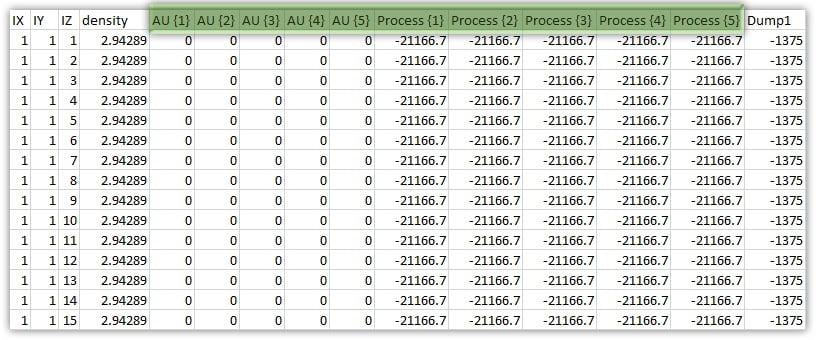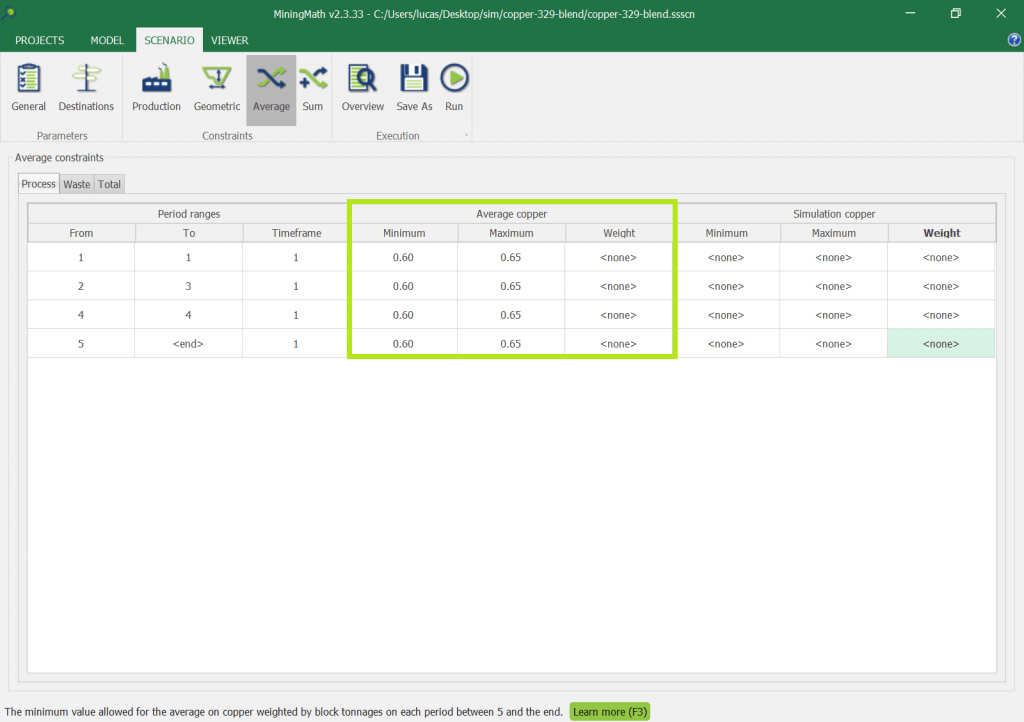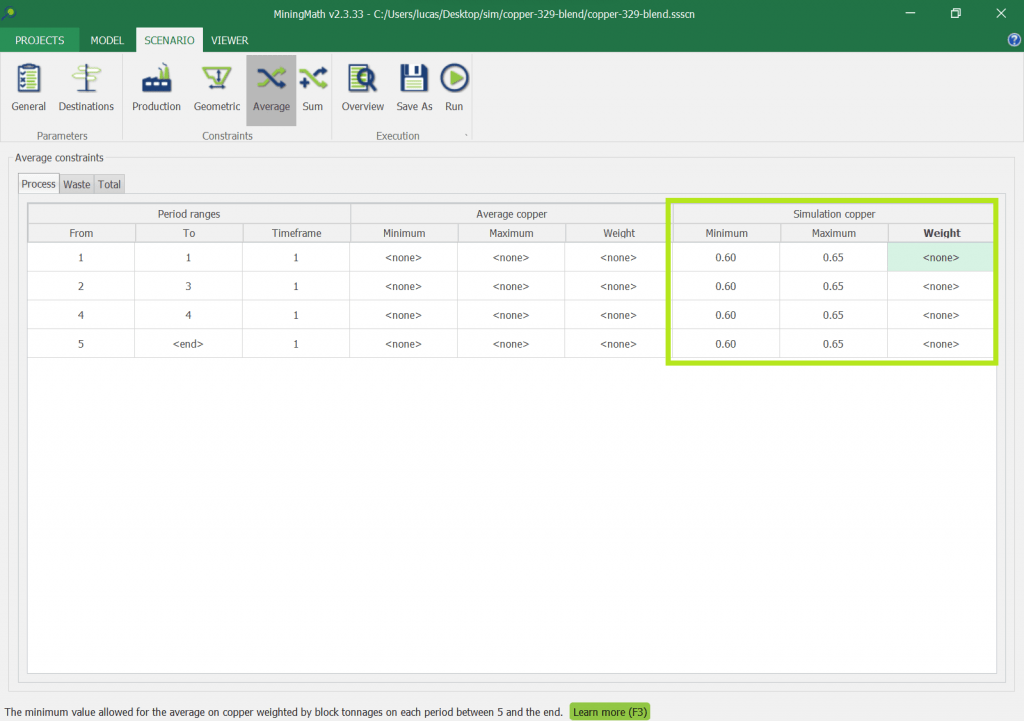#### MiningMath

Math Optimization models that integrate multiple business’ areas

# Stochastic Models

Estimated reading: 4 minutes 393 views

## Introduction

Stochastic simulations requires equiprobable models to consider uncertainties related to geological aspects, such as grade and/or volume of ore.

While single scenarios of distinct models are run separately, a stochastic scenario consist of obeying all the single scenarios at once. This is achieved through an adapted resource block model that contains equiprobable values for a given set of variables containing a certain level of uncertainty.

As a consequence, MiningMath produces reports with a risk-profile presenting the percentiles P10 and P90. These are probabilities of 10% and 90%, respectively, minimum and maximum values and an expected value.

The purpose of this page is to briefly explain how to import data and manage stochastic constraints using MiningMath. Stochastic mine planning is a wide topic and relates to long academic debates beyond the scope of this technical material. Please, check here, and especially here, for a deeper understanding of stochastic mine planning.

## Formatting

Stochastic models have specific formatting for the headers of uncertain fields. The figure below illustrates parts of the gold deposit fromFigure 1: Stochastic Values for AU and Process for 5 simulations.

Uncertain-fields are those which might vary from simulation to simulation, as explained before. Typically, grade fields contain uncertain information. Therefore, the user will need to rename each equiprobable possibility in a specific way: name each grade column as the same adding {#} (where # is a number from 1 up to n). The list below highlight how grade headers should look like:

• AU {1}

• AU {2}

• AU {3}

• AU {4}

• AU {5}

Note that, as gold is sold pure, the grade information will influence the economic values for the processing stream. Therefore, the user will need to calculate the Economic Values for each possible grade information, as highlighted in Figure 1.

Once you import your stochastic block model, the tabs Average and Sum will allow for constraints both on:

1. Expected values to control the averages over all simulations.

These constraints will guarantee that, in average, the indicators will be within the defined ranges. For example, take Expected Min = 0.60 and Expected Max = 0.65 for a certain constraint. If there are 3 simulations returning 0.59, 0.62 and 0.65, the average is 0.62, so this is within the range defined.Example to control the average of all simulations. This option is only available when databases containing stochastic data are imported.
2. All simulations to guarantee that each one of them respect certain criteria

These constraints control the variability, or the spread, of the results to be within a certain acceptable range. Let's take an example where such a range has Min = 0.60 and Max = 0.65, and again three simulations returning 0.59, 0.62, and 0.65. In this case the solution will be penalized by the optimizer, as 0.59 < 0.60. Learn more about penalized solutions here.Example to control all simulations individually. This option is only available when databases containing stochastic data are imported.

.

Stochastic optimization is an optimized way to combine all these modelled uncertainties into one schedule that maximizes the Expected NPV of the project.

## Why average NPV (single and distinct scenarios) is greater than expected NPV (stochastic scenario)?

Running different scenarios individually, the optimizer will find the best result possible for each one.

Running a stochastic one, the optimizer will find the best solution that considers all assumptions. Therefore, the expected NPV will be reduced in comparison with the average NPV from the first approach because it is more close to the reality.

## Imperial System

For importing databases, MiningMath uses the metric system exclusively. In case ...

## In-depth MiningMath

This tutorial provides a detailed guidance to the pages in the knowledge base fo...

## Theory Validation

MiningMath’s results are only possible due to its proprietary Math Program...

## Guaranteed Solutions

Multiple, complex constraints increase the likelihood of not finding or not exis...

## MiningMath Uniqueness

MiningMath allows mining managers to improve their strategic analysis through ri...

## Time Limit

It is possible to indicate a time limit in hours before running a scenario in th...

## Must read articles

In order to take the maximum of MiningMath’s Optimization we recommend this fl...

## Formatting the Block Model

The main focus here is on the requirements. Try to pay attention to the header...